# 什么是数组

• 连续的内存空间和相同的数据类型

Java 中的 ArrayList 集合类，底层就是使用数组实现的，虽然我们不需要手动进行扩容，但是一旦超过了定义的存储范围，触发扩容操作时，ArrayList 底层就是通过 Arrays.copyOf 的方式进行的扩容：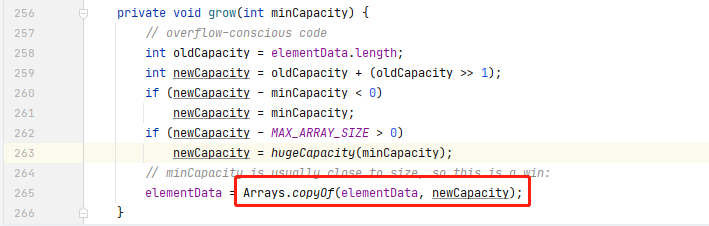Arrays.copyOf 方法就会申请一个新数组来存储旧数组的元素。正是因为数组的这种扩容方式会带来性能上的消耗，所以当我们使用 ArrayList 时，如果可以预判长度，最好指定一个长度（HashMap 也是同理），这样可以避免后期触发扩容。

• 线性表结构

## 数组的初始化

Java 中可以通过以下方式定义一个数组：

int arr[] = new int;
int[] arr1 = new int;


int[] arr3 = new int[]{1,2,3};


 int[] arr4 = {1,2,3,4};


## 单调数组

• 单调递增数组：对于所有 i <= j，有 A[i] <= A[j]

• 单调递减数组：对于所有 i <= j，有 A[i] >= A[j]

## 区分 length 和 size

int[] arr = new int;
arr = 1;


public static void main(String[] args) {
List list = new ArrayList<>(16);
System.out.println(list.size());//输出 1
}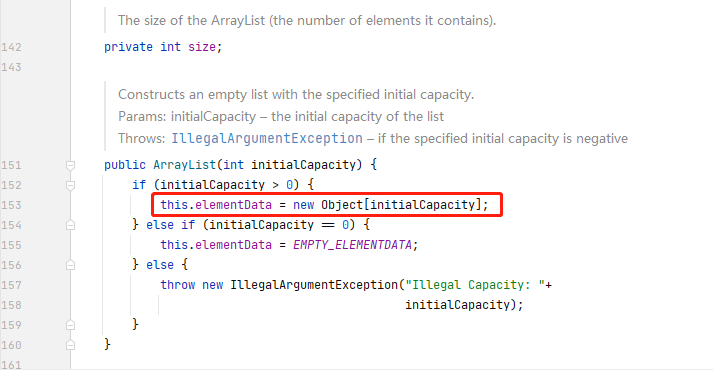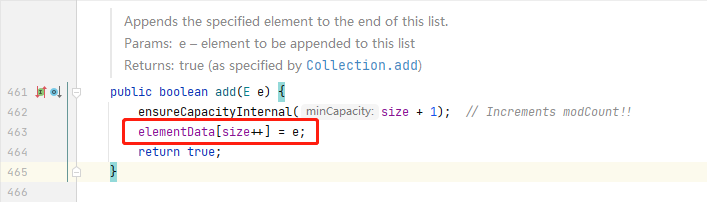# 数组的增删改查

## 访问数组元素

/**
* 寻找指定元素在数组中的下标
* @param arr - 指定数组
* @param element 要查找的元素
* @return
*/
public static int findIndexByElement(int[] arr, int size, int element) {
for (int i=0;i<size;i++){
if (arr[i] == element){
return i;
}
}
return -1;
}


## 删除数组元素

/**
* 移除数组中指定下标的元素。将 index 之后的元素往前移动一位，同时更新size即可
* @param arr - 指定数组
* @param size - 当前数组元素数量
* @param index 删除位置
* @return
*/
public static void removeByIndex(int[] arr, int size, int index) {
if (null == arr || arr.length == 0){//数组是否为空
return;
}
if (index < 0 || index > size -1){//判断是否越界
return;
}
for (int i = index;i < size;i++){//从 index 开始，所有的元素都往前移动一位
arr[i-1] = arr[i];
}
size--;//注意要维护 size
}


• 数组的边界控制
• size 的维护

## 插入数组元素

public static void main(String[] args) {
int[] arr = new int;
arr = 1;
}


• 方法一：根据元素找到需要插入的位置，然后执行插入操作，插入的同时，其他元素都往后移动。
• 方法二：直接从后往前开始比较，如果比较的结果比插入元素大，那么往后移动一位，直到找到自己的插入位置。

/**
* 将给定的元素插⼊到单调递增数组中
* @param arr - 指定数组
* @param size - 数组已经存储的元素数量
* @param element - 待插入的元素
* @return
*/
public static void addByElementSequence2(int[] arr, int size, int element) {
if (null == arr || arr.length == 0){//数组是否为空
return;
}
if (size >= arr.length){ //确认数组至少有一个空位
return;
}
boolean succ = false;
for (int i= size -1;i>=0;i--){
if (arr[i] > element){//如果当前元素大于插入元素，那么将元素后移
arr[i+1] = arr[i];//这里不会越界是因为方法中的第二个判断确保了数组至少有一个空位
}else{//如果当前元素小于等于插入元素，那么可以插入
arr[i+1] = element;//i 后面一个位置已经空出来了
succ = true;
break;
}
}
if (!succ){//如果上面没有插入成功，那就说明当前插入的元素最小，直接插入头部即可
arr = element;
}
}


 /**
* 使用 O(1) 时间复杂度在给定数组的指定位置插入元素，可以忽略顺序
* @param arr - 指定数组
* @param size - 数组中有效元素
* @param index - 指定插入下标
* @param element - 指定插入元素
*/
public static void addByElement(int[] arr, int size, int index,int element) {
if (null == arr || arr.length == 0){//数组是否为空
return;
}
if (size >= arr.length){//确认数组至少有一个空位
return;
}
arr[size] = arr[index];//将 index 和有效数组位数的最后一位交换
arr[index] = element;
}


# 双指针思想

• 方法一：定义新数组来存储，空间复杂度为 O(n)

• 方法二：双重循环遍历法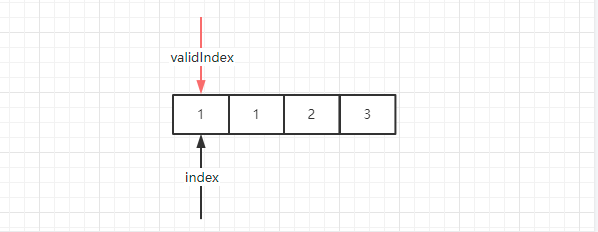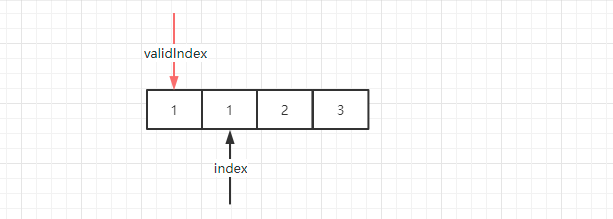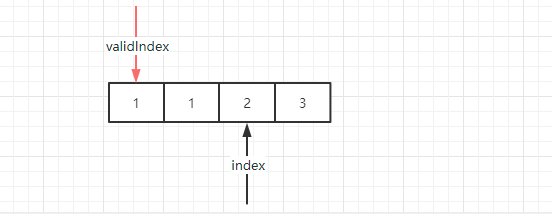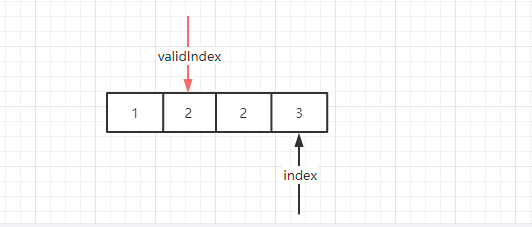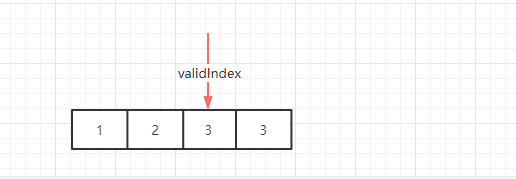/**
* 删除数组中的重复元素
* @param arr - 数组
* @return 返回剩余数组新长度
*/
public static int removeRepeatElement(int[] arr) {
if (null == arr || arr.length == 0){
return -1;
}

int validLength = 1;//有效长度
for (int i=0;i<arr.length;i++){
if (arr[i] != arr[validLength - 1]){
arr[validLength++] = arr[i];
}
}
return validLength;
}


# 总结

posted @ 2021-12-24 22:06  双子孤狼  阅读(316)  评论(0编辑  收藏  举报